# 978-0123869449 Chapter 6 Part 1

Document Type
Homework Help
Book Title
Authors
Michael F. Modest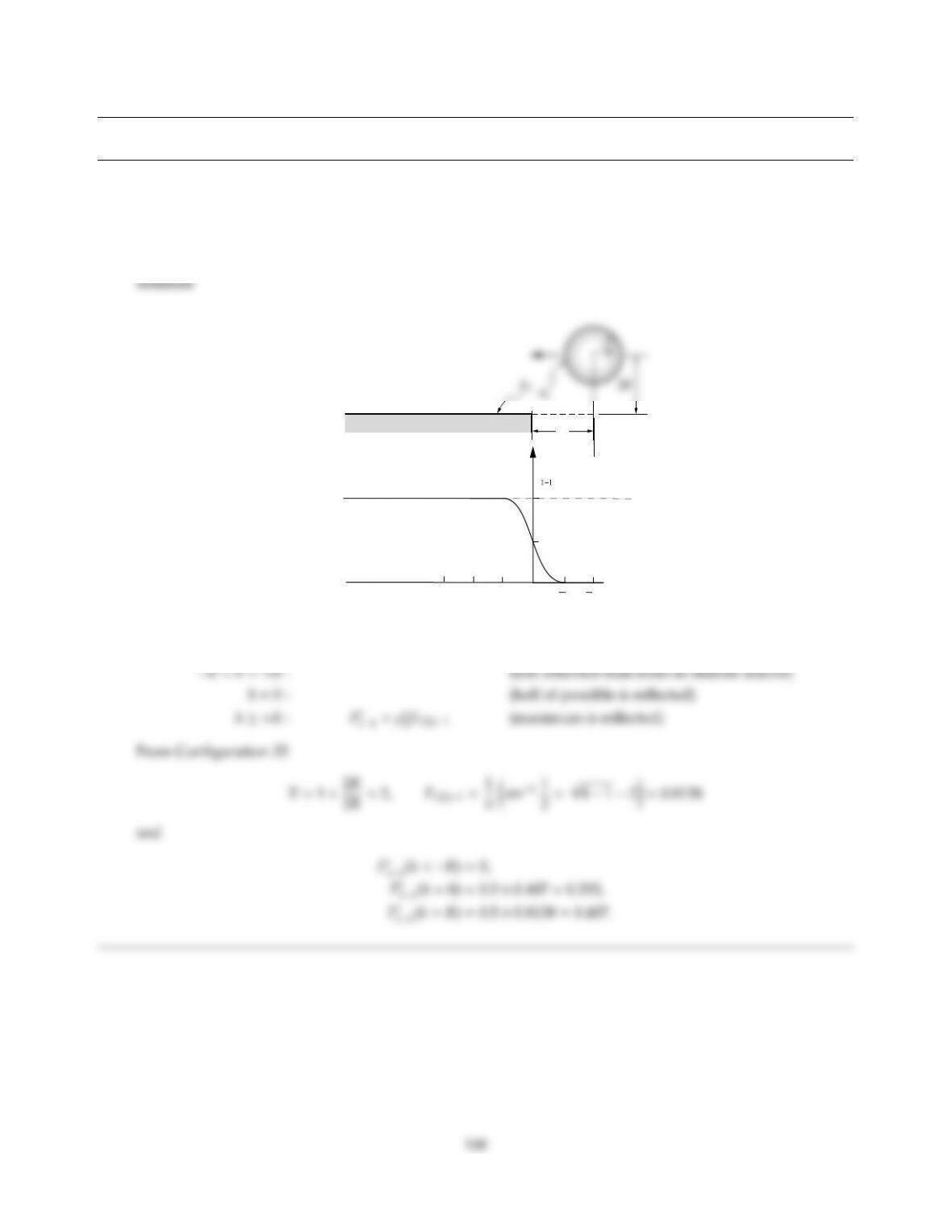CHAPTER 6
6.1 An infinitely long, diusely reflecting cylinder is opposite a large, infinitely long plate of semiinfinite width
(in plane of paper) as shown in the adjacent sketch. The plate is specularly reflecting with ρs
2=0.5. As the
center of the cylinder moves from x= +to x=−∞ plot Fs
11vs. position h(your plot should include at least
3 precise values).
h
3R 2R R 0 R 2R
Fs
0.0203
0.0407
h≤ −R:Fs
11=0 (none of radiation leaving 1 bounces back)CHAPTER 6 169
6.2 Consider two identical conical cavities (such as the ones depicted next to Problems 6.7 and 6.8), which are
identical except for their surface treatment, making one surface a diuse and the other a specular reflector. If
both cones are isothermal, and both lose the same total amount of heat by radiation, which one has the higher
temperature?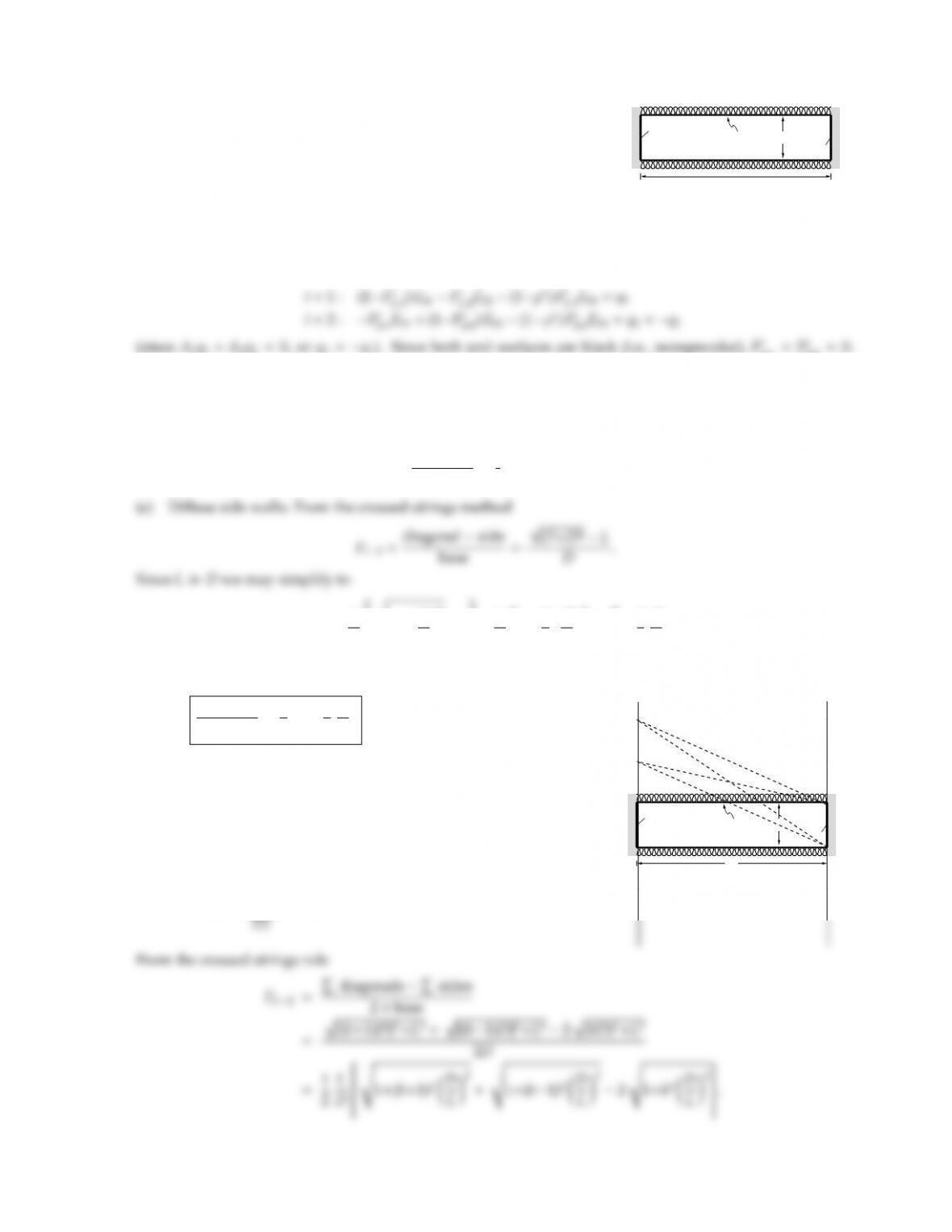6.3
L
DT2
T1
A1 A2
Two infinitely long black plates of width Dare separated by a long,
narrow channel, as indicated in the adjacent sketch. One plate is
isothermal at T1, the other is isothermal at T2. The emittance of
the insulated channel wall is ǫ. Determine the radiative heat flux
between the plates if the channel wall is (a) specular, (b) diuse. For
simplicity you may treat the channel wall as a single node. The diuse case approximates the behavior
of a light guide, a device used to pipe daylight into interior, windowless spaces.
Solution
From equation (6.22), with ǫ1=ǫ2=1 and q3=0, Hoi =0,
11=Fs
22=0.
Also, from symmetry Fs
13=Fs
23, and Fs
12=Fs
21. Thus, subtracting the two equations from one another (to
(1+Fs
21)Eb1(1+Fs
12)Eb2=2q1,
or
q1
σ(T4
1T4
2)=1
2(1+Fs
12).
F12=L
Ds1+D
L2
1L
D"1+1
2D
L2
1#=1
2
D
L.
Thus,
q1
σ(T4
1T4
2)=1
21+1
2
D
L
(b) Specular side walls:
Fs
12=F12+ρsF1(3a)2+F1(3b)2
+(ρs)2F1(3a3b)2+F1(3b3a)2
+. . .
=F12+2
X
(ρs)kFk2
L
DT2
T1
A1 A2
A3a
A3b
A1*=A1(3a)
A2*=A1(3b,3a)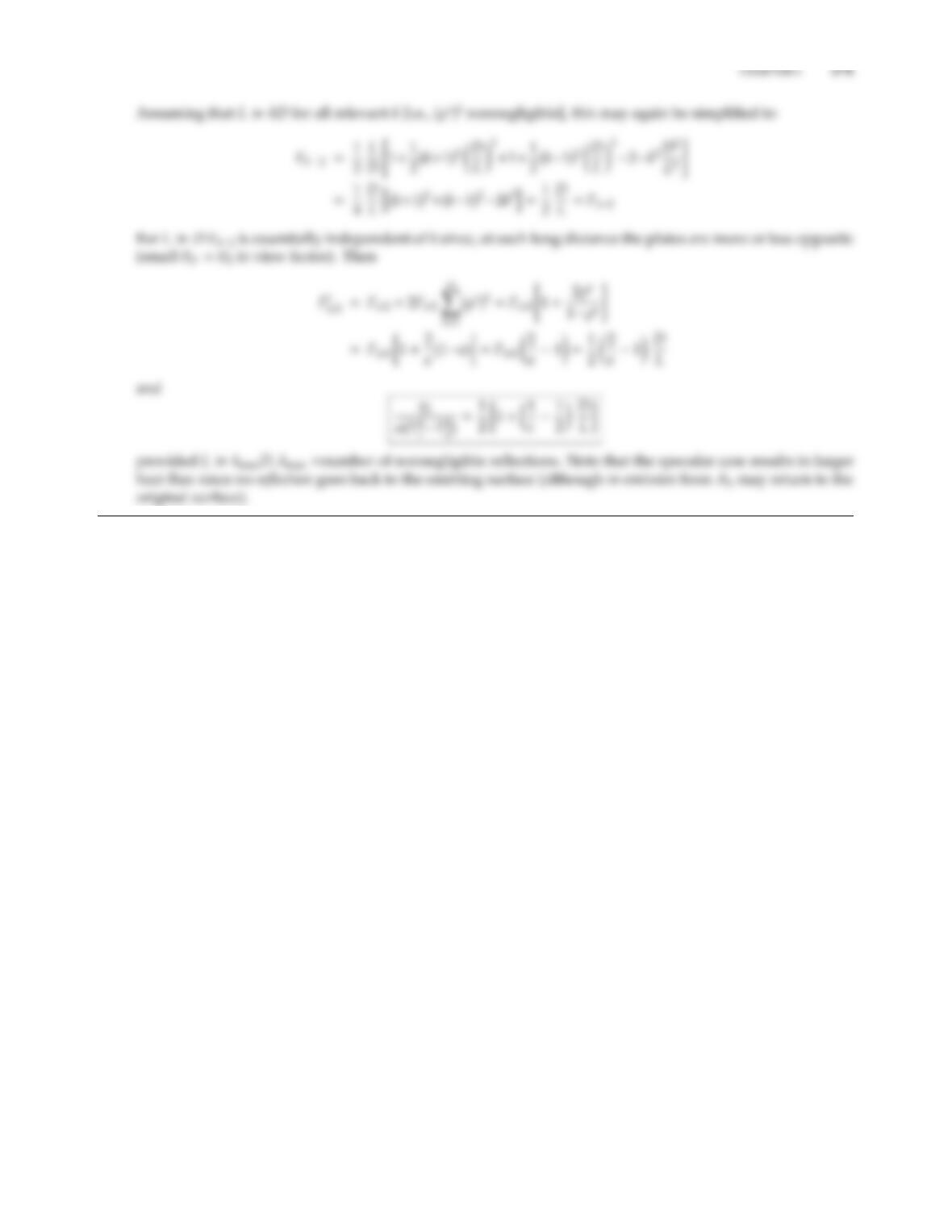6.4 Two circular black plates of diameter Dare separated by a long, narrow tubular channel, as indicated in the
sketch next to Problem 6.3. One disk is isothermal at T1, the other is isothermal at T2. The channel wall is
a perfect reflector, i.e., ǫ=0. Determine the radiative heat flux between the disks if the channel wall is (a)
specular, (b) diuse. For simplicity, you may treat the channel wall as a single node. If the channel is made of
a transparent material, the specular arrangement approximates the behavior of an optical fiber; if the channel
is filled with air, the diuse case approximates the behavior of a light guide, a device used to pipe daylight
into interior, windowless spaces.
Solution
From equation (6.22), with ǫ1=ǫ2=1, Hoi =0, and q3=0 (since the wall neither emits nor absorbs radiation),
i=1 : (1Fs
11)Eb1Fs
12Eb2(1ρs)Fs
13Eb3=q1
i=2 : Fs
21Eb1+(1Fs
22)Eb2(1ρs)Fs
23Eb3=q2=q1
(since A1q1+A2q2=0, or q2=q1). Since both end surfaces are black (i.e., nonspecular), Fs
11=Fs
22=0.
Also, from symmetry Fs
13=Fs
23, and Fs
12=Fs
21. Thus, subtracting the two equations from one another (to
(1+Fs
21)Eb1(1+Fs
12)Eb2=2q1,
or
q1
σ(T4
1T4
2)=1
2(1+Fs
12).
(a) Diuse side wall: from Appendix D, Configuration 40,
D
="1+2L
D2#
1s1"1+2L
D2#2
.
For L/D1 this becomes (with 1+x1+1
2x x 1)
F12"1+2L
11+1
regardless of the side wall’s emittance.
(b) Specular side wall: Recalling the definition of a specular view factor, equation (6.3), it is clear that
Fs
12=Fs
21=1 since (i) all diuse energy leaving A1(i.e., emission) is intercepted by A2(since, because of theCHAPTER 6 173
specular side wall, nothing is reflected back to A1, and nothing is absorbed by the side wall), (ii) every light
ray hits A2only once (black wall), eliminating multiple contributions (see, e.g., Example 6.1). This can also
be shown mathematically: Setting ρs=1, the two renditions of equation (6.22) become
which is, of course, why optical fibers work. Note that the above arguments allow the fiber to be nonstraight,
i.e., twisted to any arbitrary degree.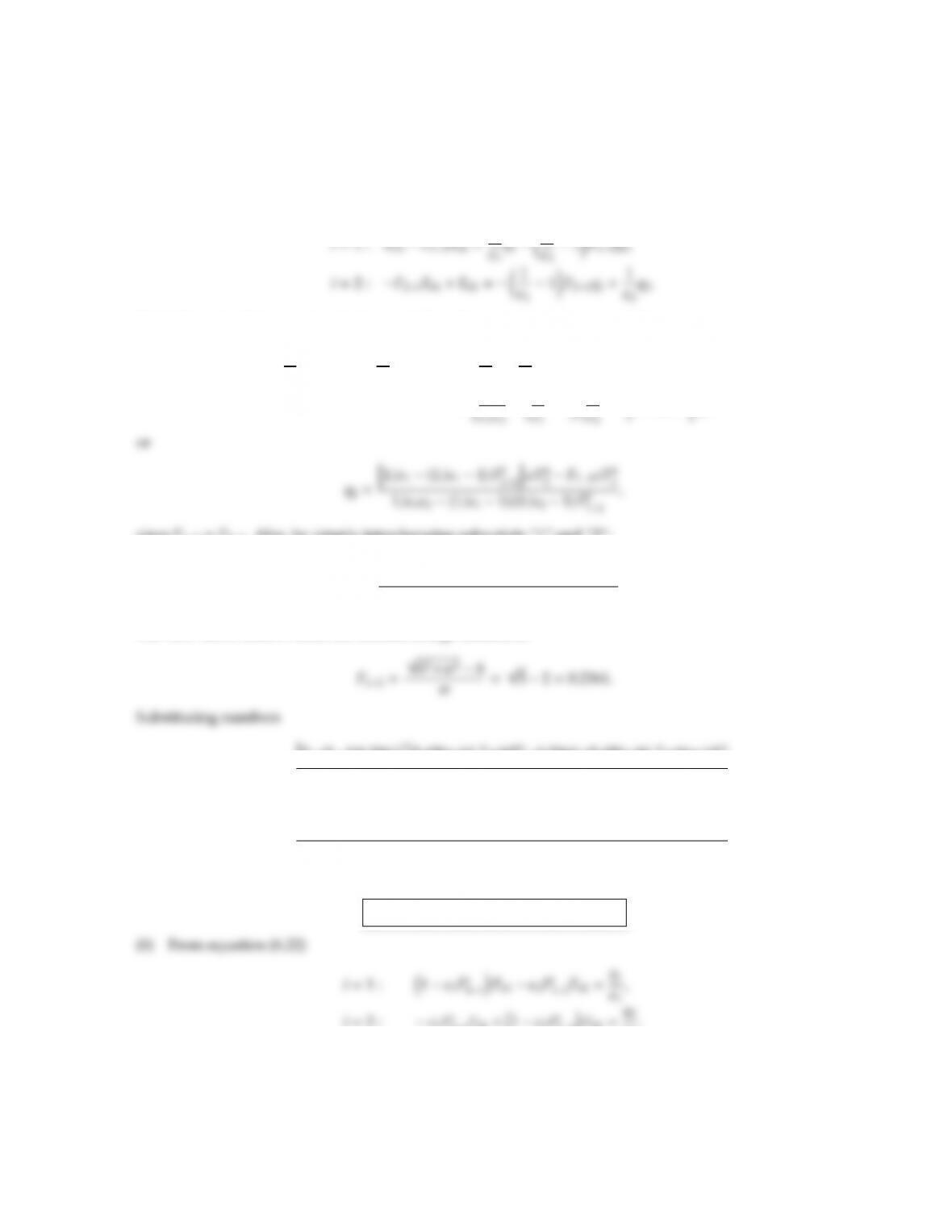6.5 Two infinitely long parallel plates of width ware spaced h=2wapart. Surface 1 has ǫ1=0.2 and T1=1000 K,
Surface 2 has ǫ2=0.5 and T2=2000 K. Calculate the heat transfer on these plates if (a) the surfaces are diuse
reflectors, (b) the surfaces are specular.
Solution
(a) From equation (5.37), with Hoi =0,
Multiplying the first equation by (111)F21, the second by 11, and adding
1
ǫ11F211
ǫ1
F21Eb1+1
ǫ11
ǫ11F21F12Eb2
=1
since F12=F21. Also, by simply interchanging subscripts “1” and “2”:
q1=h12(121)F2
12iσT4
1F12σT4
2
11ǫ2(111) (121)F2
12
.
The view factor follows from the crossed strings method as
q1=h2(21)0.23612i5.670×108×1012 0.2361×5.670×108×16×1012
10 (51)(21) ×0.23612
=10,629 W/m2,
q2=h5(51)0.23612i5.670×108×16×1012 0.2361×5.670×108×1012
10 (51)(21) ×0.23612
=441,890 W/m2.
q1=1.06 W/cm2,q2= +44.19 W/cm2
i=2 : ǫ1Fs
21Eb1+1ǫ2Fs
22Eb2=q2
ǫ2
.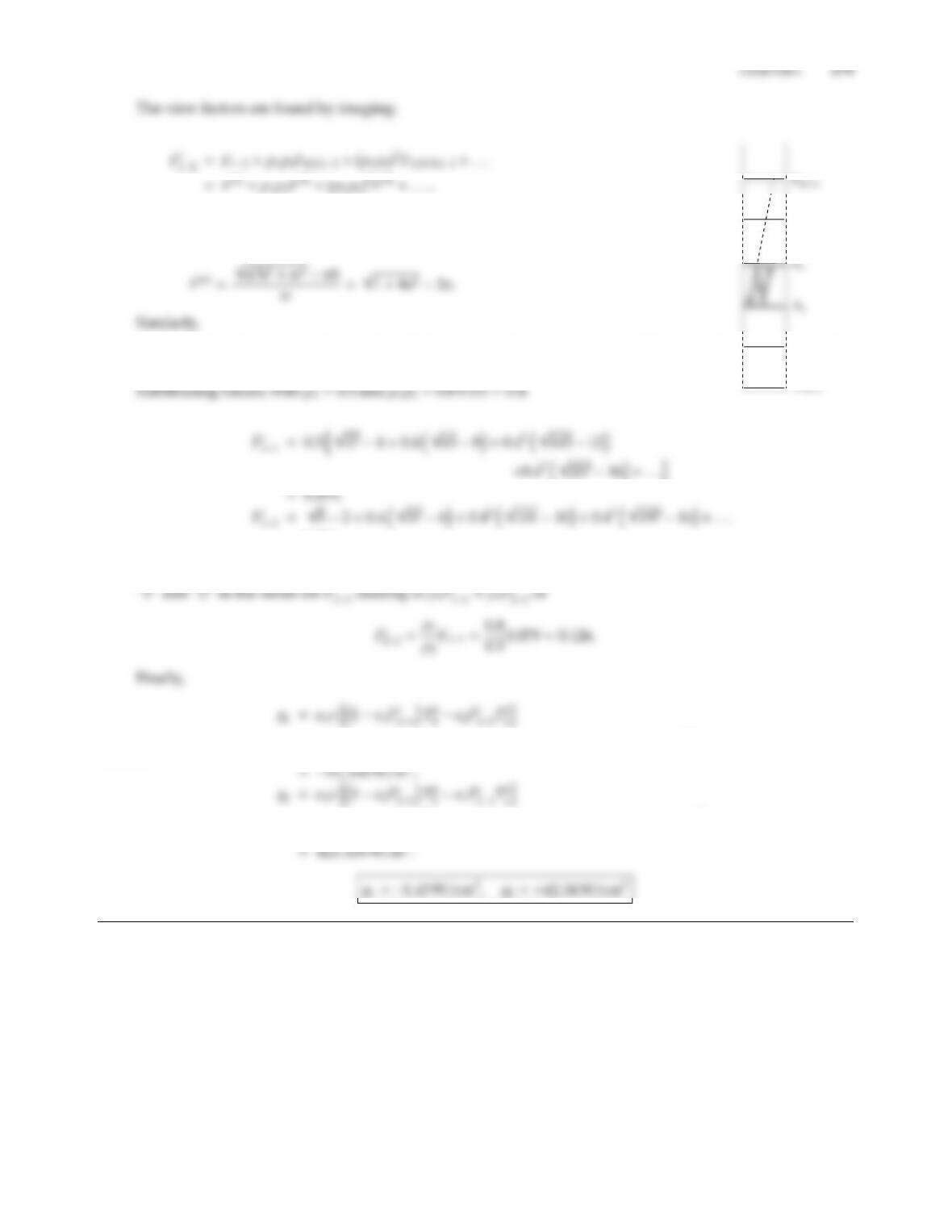where F(n)is the view factor between plates spaced n×h
apart, i.e.,
Fs
11=ρ2F(2) +ρ1ρ2
2F(4) +ρ2
1ρ3
2F(6) +. . .
A1(2)
A2(1)
A1(21)
=0.281.
Using reciprocity, Fs
21follows directly from Fs
21=Fs
12=0.281. Fs
22may be found by switching subscripts
=0.2×5.670×108h(10.2×0.079)1012 0.5×0.281×16×1012i
=0.5×5.670×108h(10.5×0.126)×16×1012 0.2×0.281×1012i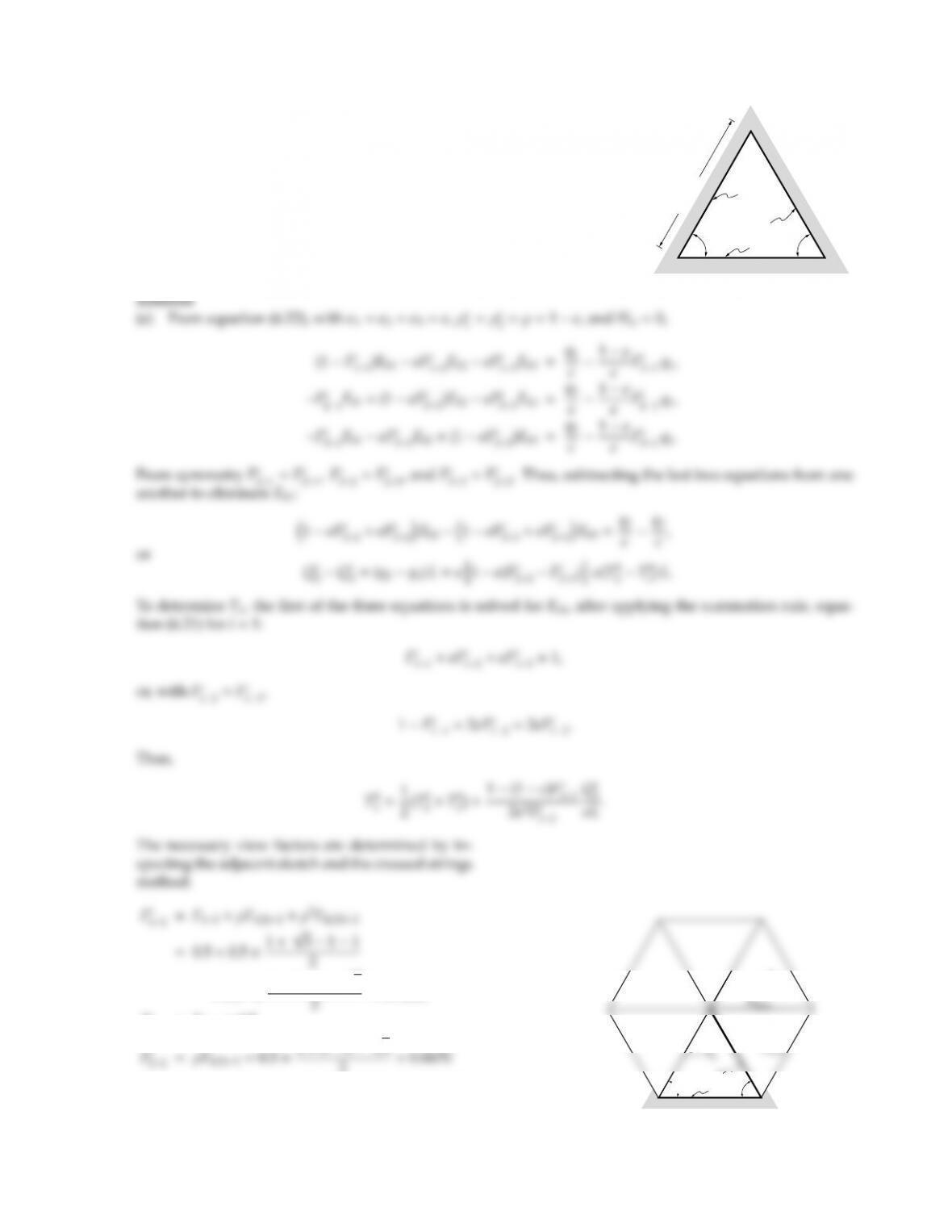6.6
60°
A3
A2
A1
60°
L = 1 m
A long duct has the cross-section of an equilateral triangle with side
lengths L=1 m. Surface 1 is a diuse reflector to which an external heat
flux at the rate of Q
1=1 kW/m length of duct is supplied. Surfaces 2
and 3 are isothermal at T2=1000 K and T3=500 K, respectively, and are
purely specular reflectors with ǫ1=ǫ2=ǫ3=0.5.
(a) Determine the average temperature of Surface 1, and the heat fluxes
for Surfaces 2 and 3.
(b) How would the results change if Surfaces 2 and 3 were also diusely
reflecting?
+0.52×1+213
Fs
23=F23=0.5
2=0.0670
Fs
11=12ǫFs
12=12×0.5×0.7165 =0.2835
60°
A3
A1
60°
A1(3)
A1(23)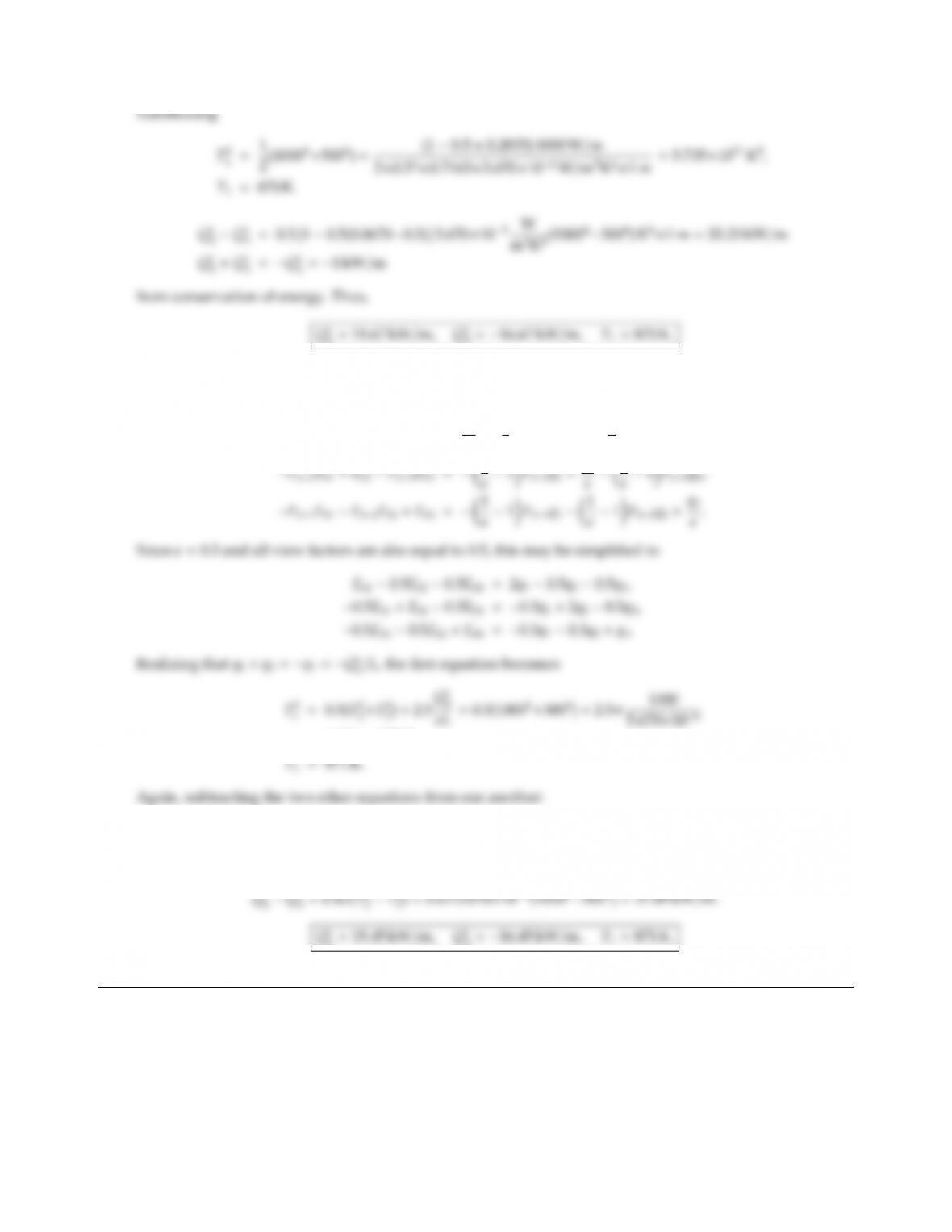CHAPTER 6 177
2=15.67 kW/m,Q
3=16.67 kW/m,T1=870 K.
(b) If all three surfaces are diuse reflectors, equation (5.36) holds, or
Eb1F12Eb2F13Eb3=q1
ǫ1
ǫ1F12q21
ǫ1F13q3,
=5.753×1011K4,
1.5(Eb2Eb3)=2.5(q2q3),
or
2=15.45 kW/m,Q
3=16.45 kW/m,T1=871 K.
The answers are virtually identical for such a regular (i.e., without extreme aspect ratio) enclosure.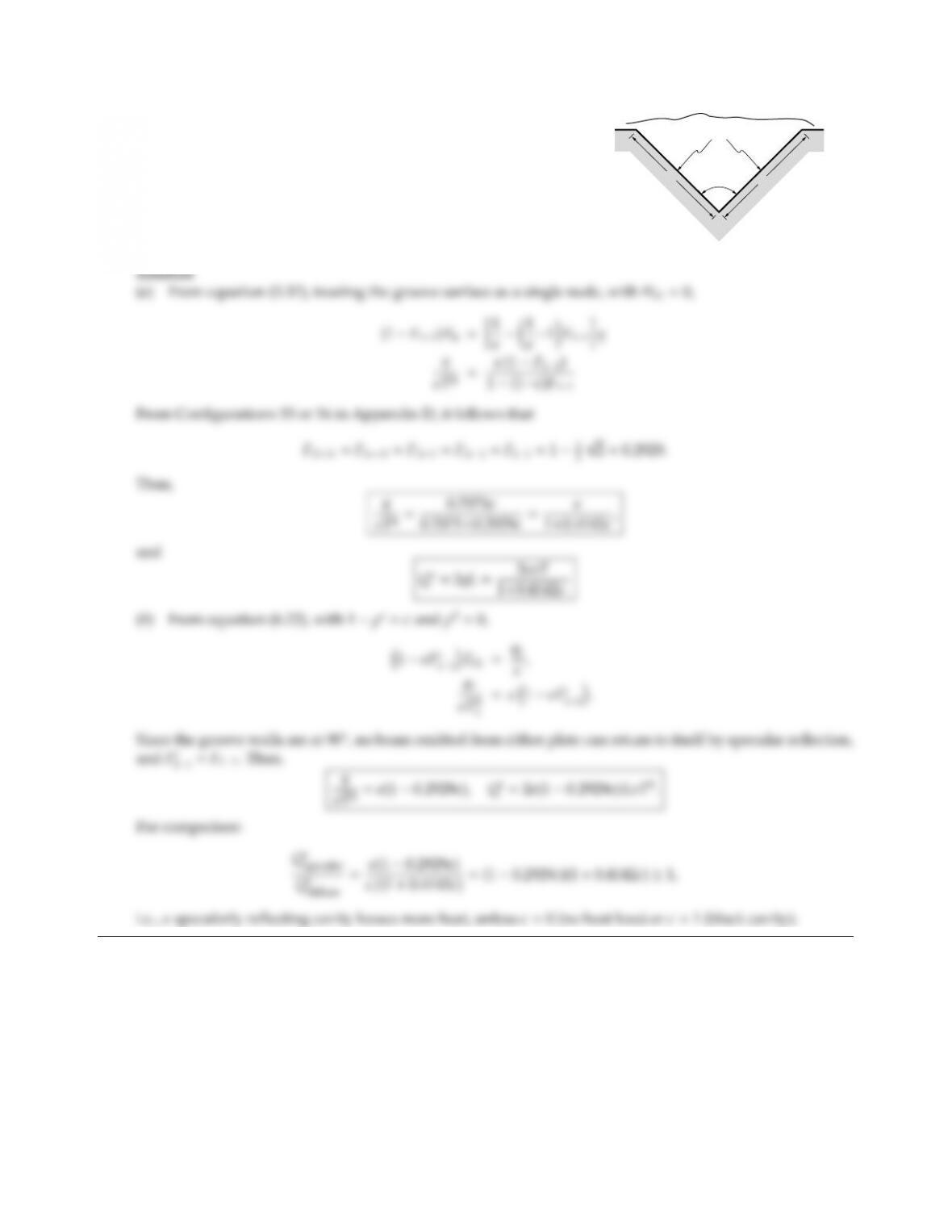6.7 Consider the infinite groove cavity shown. The entire surface of the
groove is isothermal at Tand coated with a gray, diusely emitting
material with emittance ǫ.
(a) Assuming the coating is a diuse reflector, what is the total heat
loss (per unit length) of the cavity?
(b) If the coating is a specular reflector, what is the total heat loss for
the cavity?
90o
T,
L
0 K
L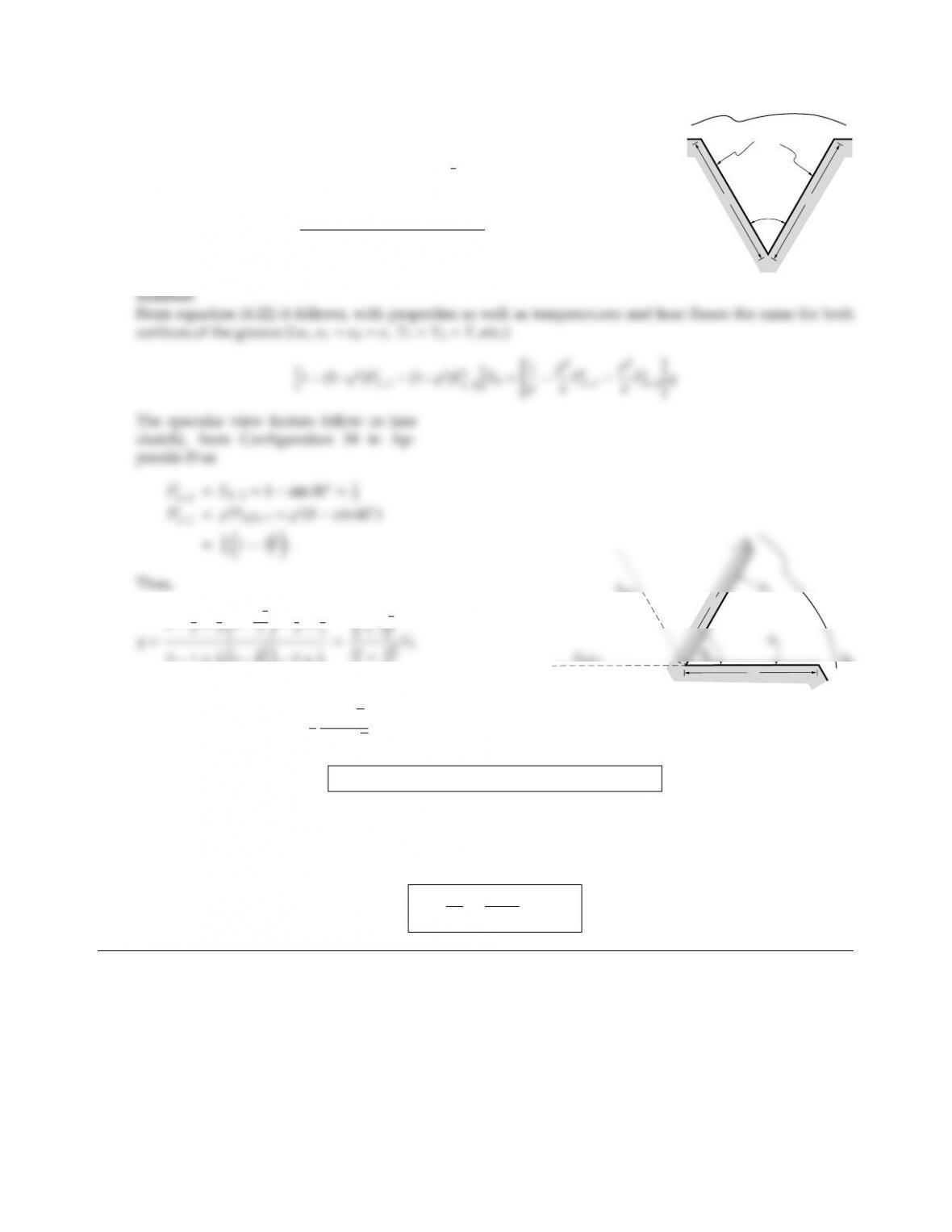CHAPTER 6 179
6.8
60o
T,
0K
LL
Consider the infinite groove cavity shown in the adjacent sketch. The entire
surface (L=2 cm) is isothermal at T=1000 K and is coated with a gray
material whose reflectance may be idealized to consist of purely diuse and
specular components such that ǫ=ρd=ρs=1
3. What is the total heat loss
from the cavity? What is its apparent emittance, defined by
ǫa=total flux leaving cavity
area of groove opening ×Eb
?
12
31×1
313
21×1
2
4
6+3
6
LL
q=2
3
4+3
13+3Eb=0.2594 ×5.670 ×1012 ×10004=1.4707 W/cm2
Q=2Lq =2×2×1.4707 =5.883 W/cm length.
On the other hand, a black plate at the same temperature, covering the opening would lose
Q
b=A
openingσT4=LσT4=2×5.670 ×1012 ×10004=11.34 W/cm,
and
ǫa=Q
Q
b
=5.883
11.34 =0.519.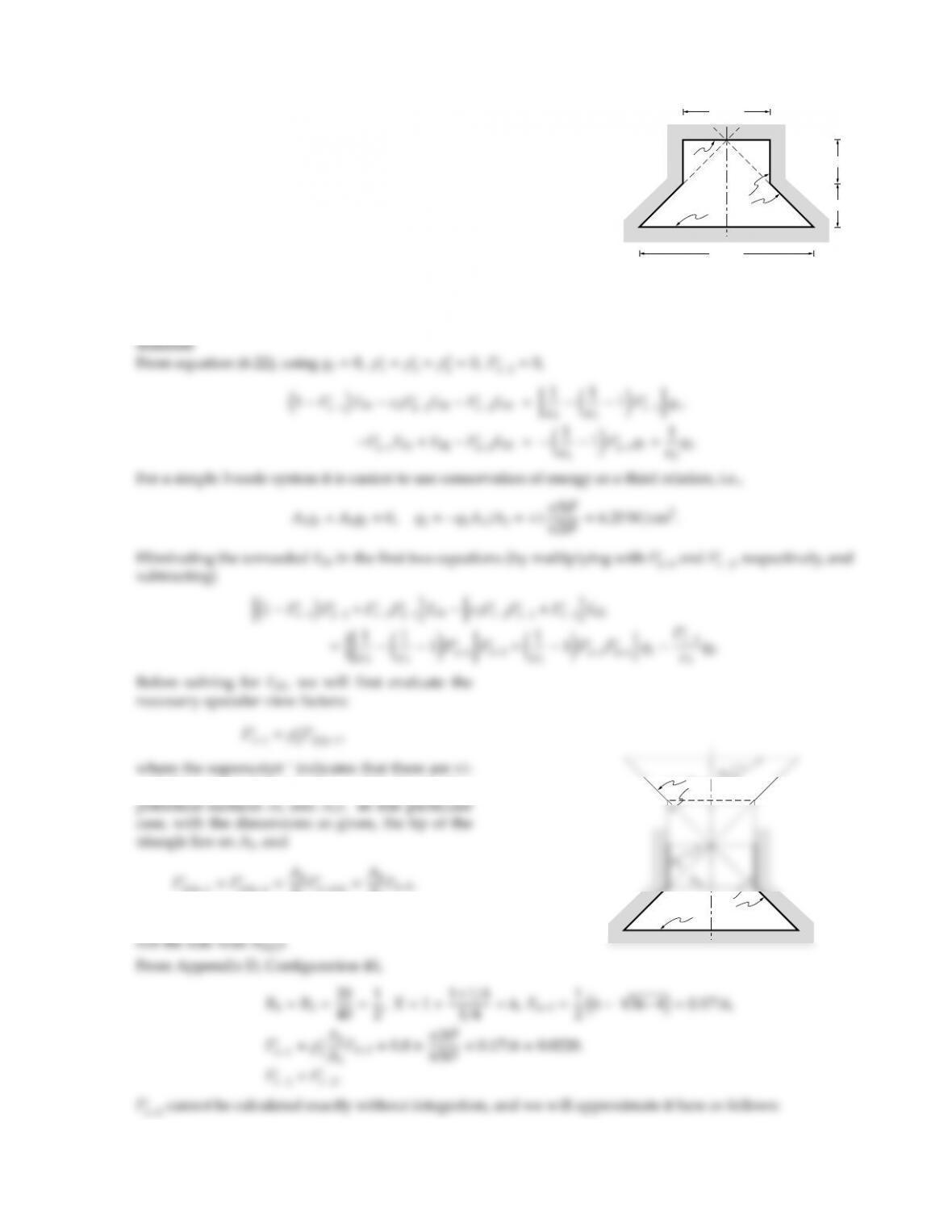6.9
10 cm
10 cm
20 cm
A2
A3
40 cm
A1
Determine the temperature of surface A2in the axisymmetric con-
figuration shown in the adjacent sketch, with the following data:
A1:T1=1000 K,q1=1 W/cm2,
ǫ1=0.6 (diuse reflector);
A2:ǫ2=0.2 (specular reflector);
A3:q3=0.0 (perfectly insulated),
ǫ3=0.3 (diuse reflector).
All surfaces are gray and emit diusely.
Note: Some view factors may have to be approximated if integration is to be avoided.
sual obstructions between A1(2) and A1(i.e., the hy-
1(2)1=F
1(2)4=A4
A1
41(2) =A4
A1
i.e., all beams from A4through A5will hit A1(2) (and
A3
A1
A3(2)
A5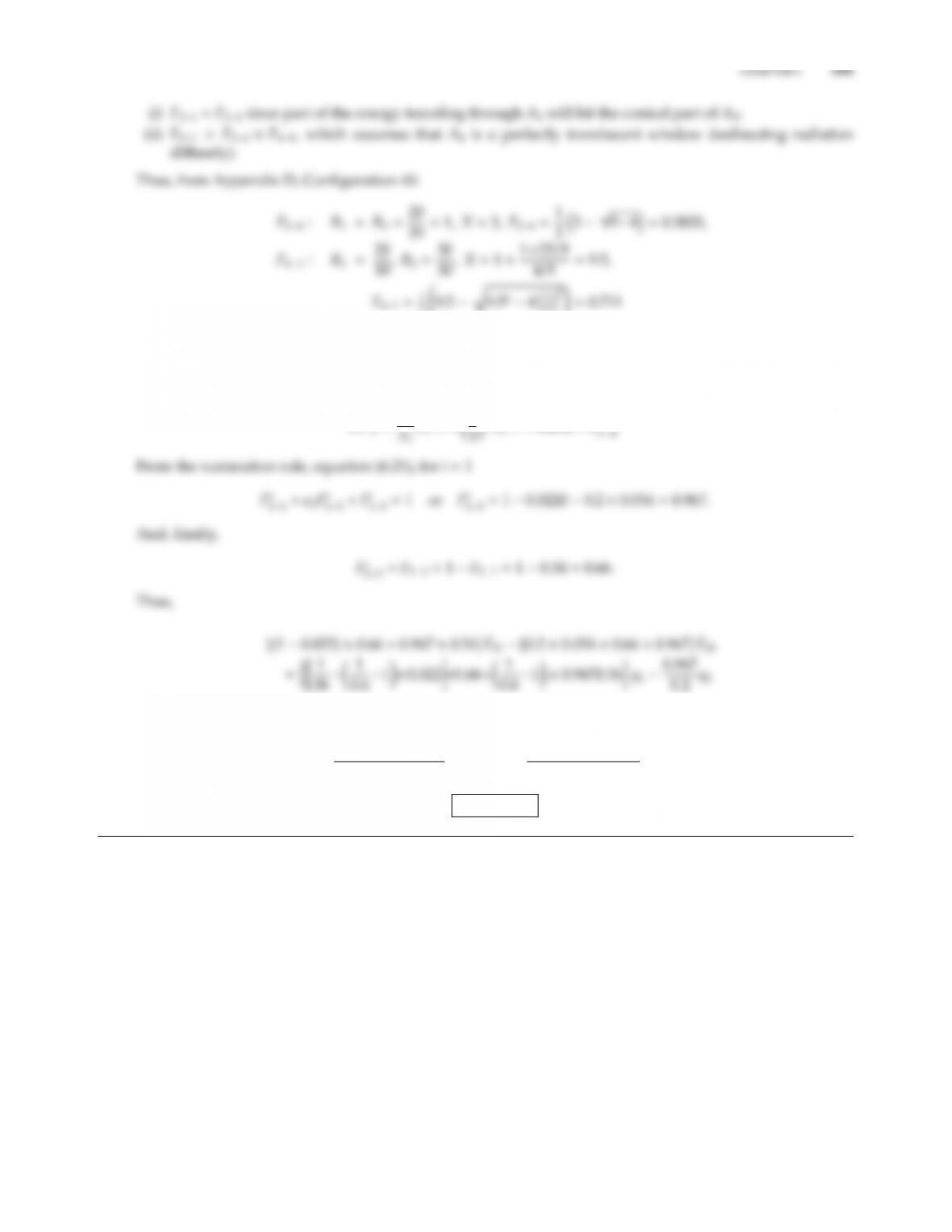2 9.5q9.5245
22!=0.711
0.3820 ×0.7111 =0.2716 <F21<0.3820 F210.34 =Fs
21.
Then
F12=A2
F21=2
F210.054 =Fs
0.974Eb10.974Eb2=1.310q14.835q2
T4
2=T4
11.310q14.835q2
0.974σ=10004+1.3101+4.8356.25
0.9745.6701012 =6.7091012
T21609 K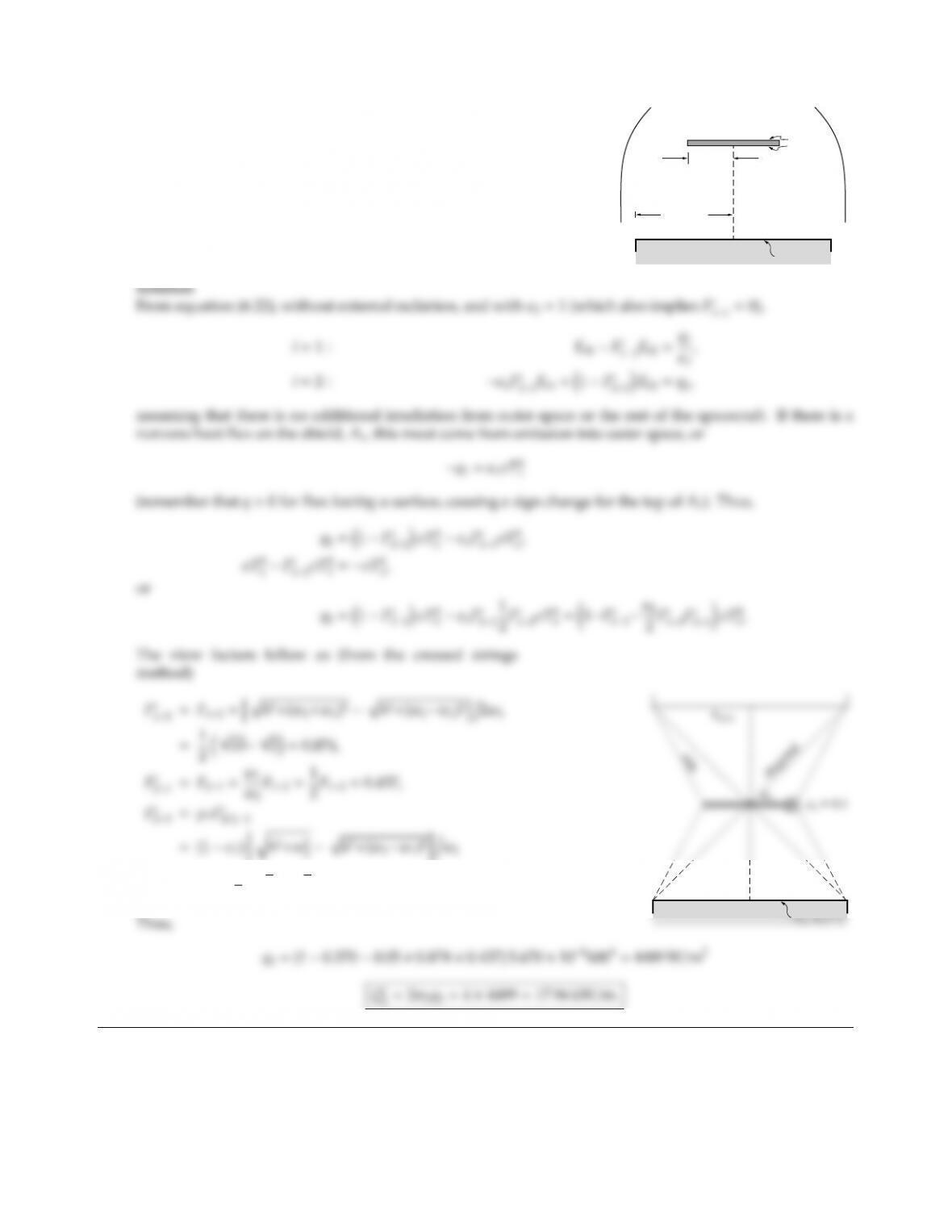6.10
w1 =1 m
h = 1 m
w2 = 2 m
T2, 2 = 1
0 K
1 = 0.1
0 K
To calculate the net heat loss from a part of a spacecraft, this part may
be approximated by an infinitely long black plate at temperature
T2=600 K, as shown. Parallel to this plate is another (infinitely
long) thin plate that is gray and reflects specularly with the same
emittance ǫ1on both sides. You may assume the surroundings to
be black at 0 K. Calculate the net heat loss from the black plate.
=0.9×1
252=0.370.
A2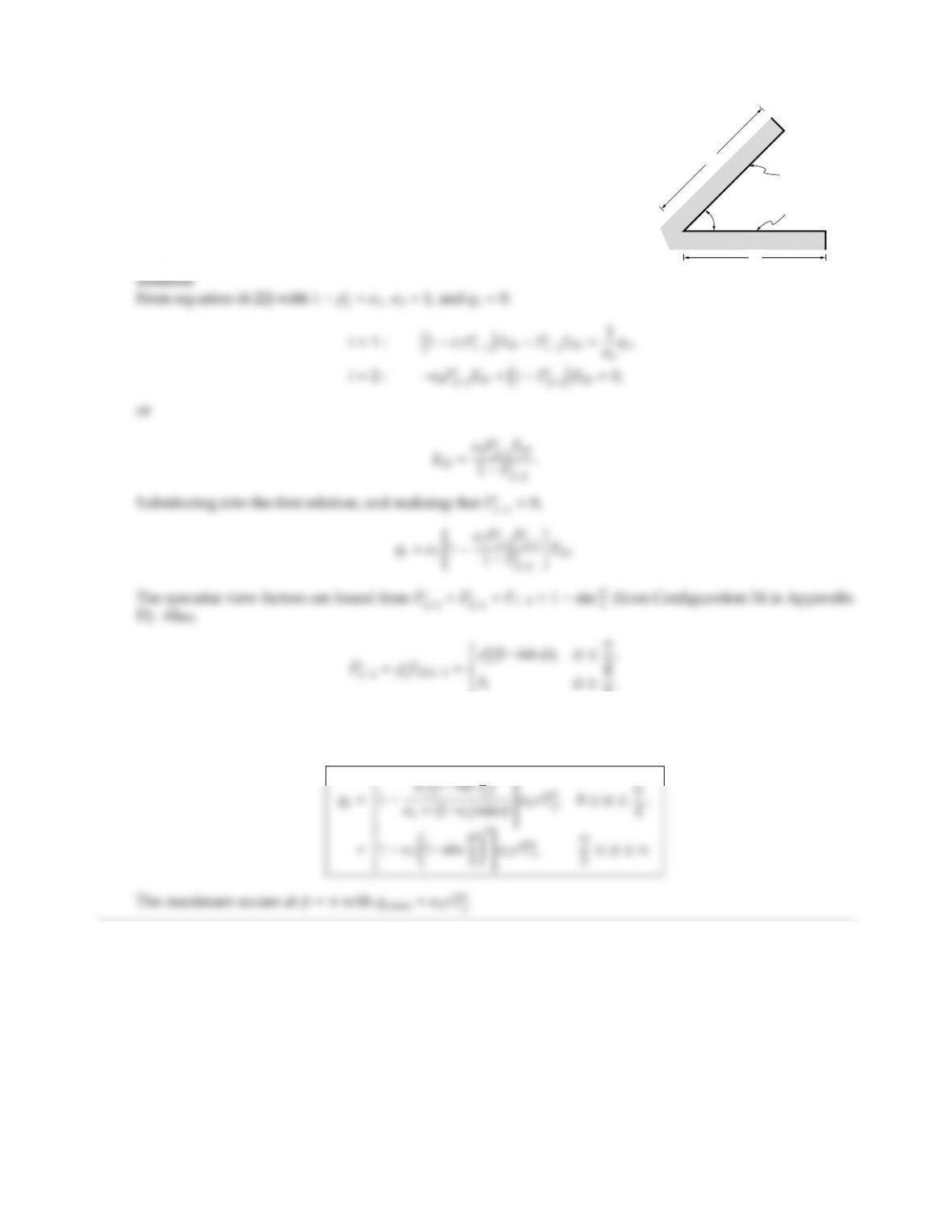CHAPTER 6 183
6.11
L
L
A2, black and
φ
T1, 1
insulated
A long isothermal plate (at T1) is a gray, diuse emitter (ǫ1) and purely
specular reflector, and is used to reject heat into space. To regulate the
heat flux the plate is shielded by another (black) plate, which is perfectly
insulated as illustrated in the adjacent sketch. Give an expression for heat
loss as function of shield opening angle (neglect variations along plates).
At what opening angle 0 φ180does maximum heat loss occur?
2.
Note that Fs
22=0 if the opening angle exceeds π/2 (unlike the expression for Fs
12, which remains valid up
to φ=π). Substituting into the relation for q1: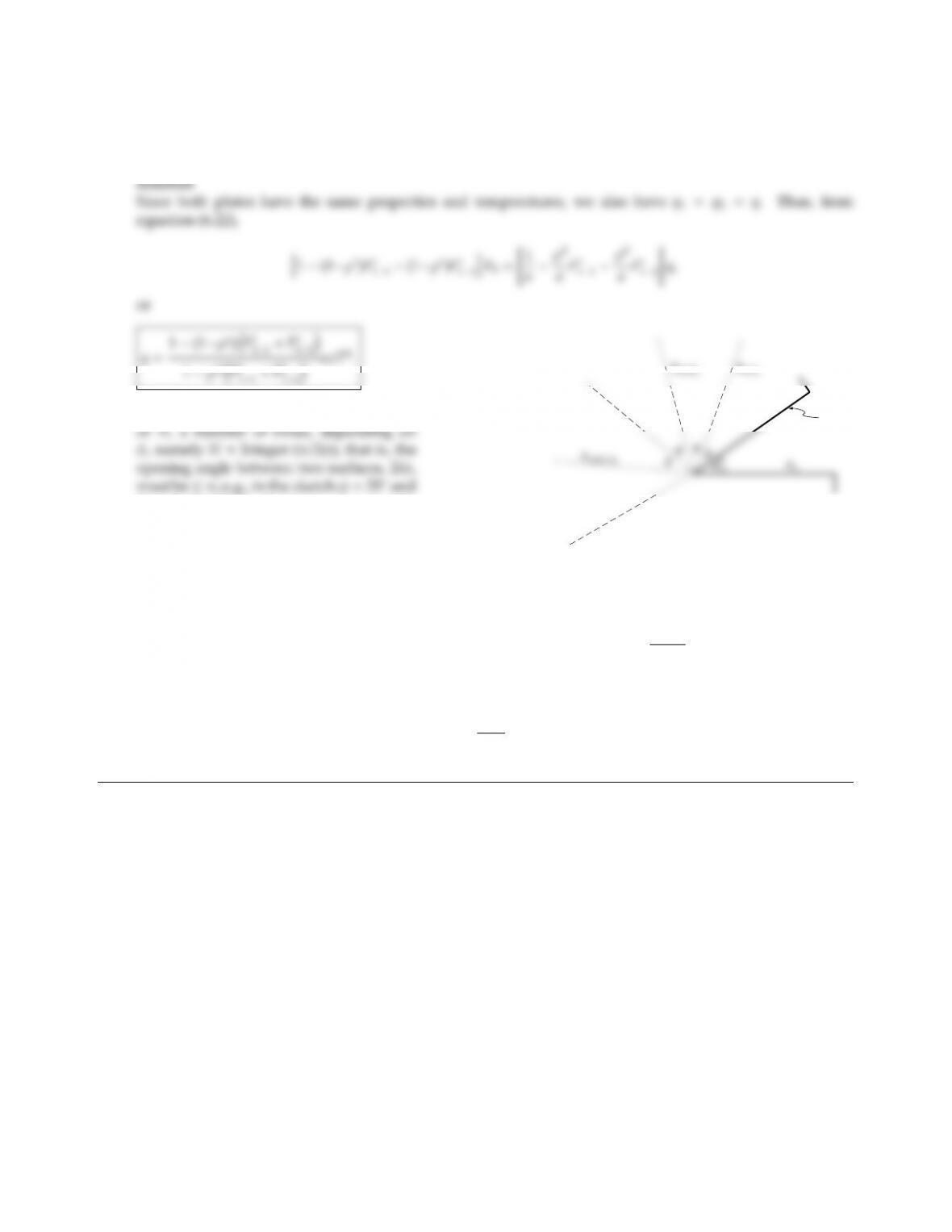6.12 Reconsider Problem 6.11, but assume the entire configuration to be isothermal at temperature T, and covered
with a partially-diuse, partially-specular material, ǫ=1ρsρd. Determine an expression for the heat lost
from the cavity.
Standing on top of A1one can see the image
N=2. Similarly, the image of A2can be
seen M=Integer (π/2φ1/2) Ntimes
(not including A2itself). Then
A2
A1(2)
A2(12)
A1(212)
Fs
11=ρsF1(2)1+(ρs)3F1(212)1+··· =
N
X
i=1
(ρs)2i1(1 sin iφ),
Fs
12=Fs
21=F21+(ρs)2F2(12)1+··· =
N
X
i=0
(ρs)2i1sin 2i+1
2φ,
or
Fs
11+Fs
12=
I
X
i=0
(ρs)i1sin i+1
2φ,I=Integer (π/φ 1).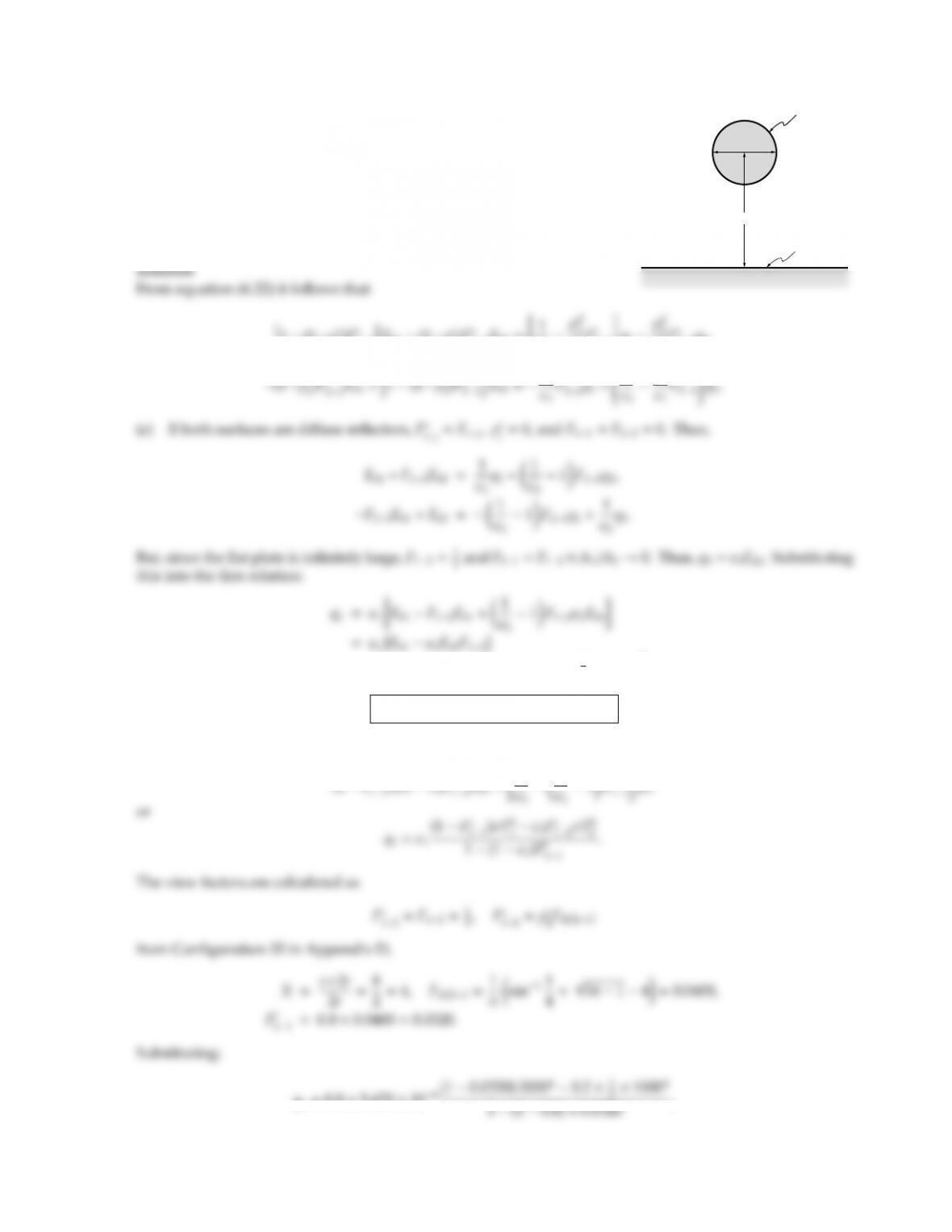CHAPTER 6 185
6.13
T2, 2
D
T1, 1
2D
An infinitely long cylinder with a gray, diuse surface (ǫ1=0.8) at
T1=2000 K is situated with its axis parallel to an infinite plane with
ǫ2=0.2 at T2=1000 K in a vacuum environment with a background
temperature of 0 K. The axis of the cylinder is two diameters from the
plane. Specify the heat loss from the cylinder when the plate surface is
(a) gray and diuse, or (b) gray and specular.
1)Fs
11iEb1(1ρs
2)Fs
12Eb2=
ǫ1ρd
ǫ1
Fs
11q1ρd
ǫ2
Fs
12q2,
1
1
2
=0.8×5.670 ×108h200040.2×1
2×10004i,
q1=7.21 ×105W/m2=72.1 W/cm2.
(b) If A2is a specular reflector, we have ρs
1=ρd
2=Fs
22=0, and
(1 Fs
q1=0.8×5.670 ×108(1 0.0320) 200040.2×1q1=7.025 ×105W/m2=70.25 W/cm2,
which shows a slight decrease from (a) since—for a specular A2—less radiation from A1is reflected back to
the cylinder.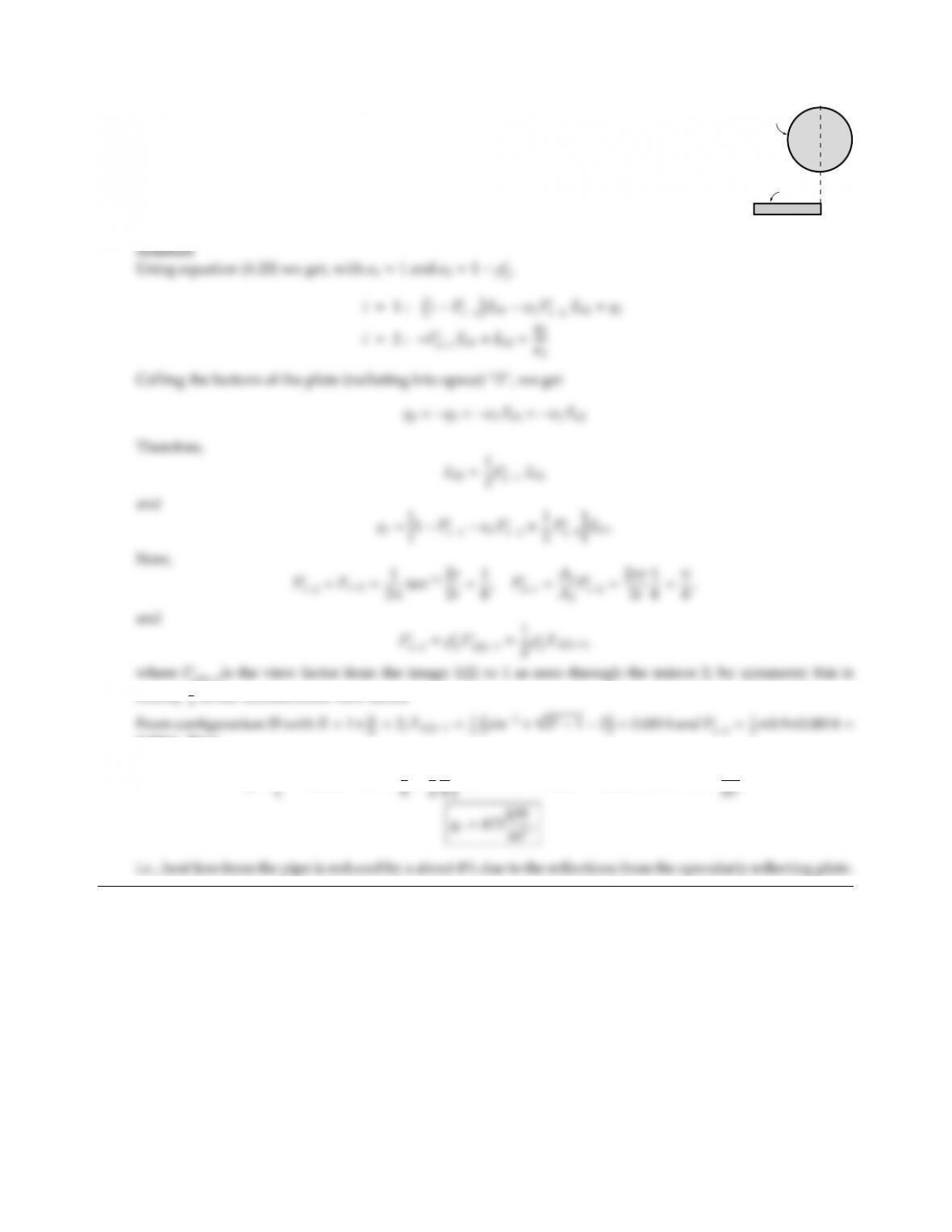CHAPTER 6 187
6.14
A1
r
2r
A2
2r
A pipe carrying hot combustion gases is in radiative contact with a thin plate as shown.
Assuming (a) the pipe to be isothermal at 2000 K and black, (b) the thin plate to be coated
on both sides with a gray, diusely emitting/specularly reflecting material (ǫ=0.1),
determine the radiative heat loss from the pipe. The surroundings are at 0 K and
convection may be neglected.
1(2)1is the view factor from the image 1(2) to 1 as seen through the mirror 2; by symmetry this is
exactly 1
0.0366. Thus
q1=10.0366 0.1×1
π

## Trusted by Thousands ofStudents

Here are what students say about us.